### SIMTESTS Statement

SIMTESTS | SIMTEST sim-test <sim-test …> ;

where sim-test represents one of the following:test-name = [ functions ]test-name = { functions } and functions are either parameters in the model or parametric functions computed in the SAS programming statements.

When the estimates in a model are asymptotically multivariate-normal, continuous and differentiable functions of the estimates are also multivariate-normally distributed. In the SIMTESTS statement, you can test these parametric functions simultaneously. The null hypothesis for the simultaneous tests is assumed to have the following form::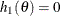,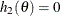, …

whereis the set of model parameters (independent or dependent) in the analysis and each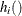is a continuous and differentiable function of the model parameters.

To test parametric functions simultaneously in the SIMTESTS statement, you first assign a name for the simultaneously test in test-name. Then you put the parametric functions for the simultaneous test inside a pair of parentheses: either the '{' and '}' pair, or the '[' and ']' pair. For example, if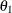,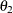,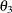, and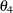are parameters in the model and you want to test the equality ofandand the equality ofandsimultaneously, you can use the following statements:

simtests
Equality_test = [t1_t2_diff t3_t4_diff];
t1_t2_diff   = theta1 - theta2;
t3_t4_diff   = theta3 - theta4;


In the SIMTESTS statement, you test two functions t1_t2_diff and t3_t4_diff simultaneously in the test named Equality_test. The two parametric functions t1_t2_diff and t3_t4_diff are computed in the SAS programming statements as differences of some parameters in the model.